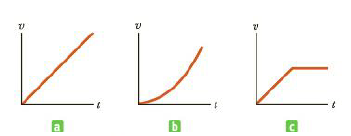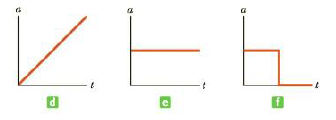Chapter 2.1, Problem 2.3QQ

Chapter
Section
Textbook Problem

Parts (a), (b), and (c) of Figure 2.10 represent three graphs of the velocities of different objects moving in straight-line paths as functions of time. The possible accelerations of each object as functions of time are shown in parts (d), (c), and (f). Match each velocity vs. time graph with the acceleration vs. time graph that best describes the motion.Figure 2.10 (Quick Quiz 2.3) Match each velocity vs. time graph to its corresponding acceleration vs. time graph.(a)

To determine
The velocity graph matching with the corresponding acceleration graph.

Explanation

Acceleration is the change in velocity. Acceleration can be found from the slope of a velocity time graph. The velocity versus time graph with constant velocity has constant acceleration.

Graph (a) represents velocity versus time

(b)

To determine
The velocity graph matching with the corresponding acceleration graph.

(c)

To determine
The velocity graph matching with the corresponding acceleration graph.

Still sussing out bartleby?

Check out a sample textbook solution.

See a sample solution

The Solution to Your Study Problems

Bartleby provides explanations to thousands of textbook problems written by our experts, many with advanced degrees!

Get Started

Calorie deficit is no longer the primary strategy for weight management.

Nutrition: Concepts and Controversies - Standalone book (MindTap Course List)

What is the nucleolus, and what is its function?

Biology: The Dynamic Science (MindTap Course List)

Most codons specify a(n) _______. a. protein b. mRNA c. amino acid

Biology: The Unity and Diversity of Life (MindTap Course List)